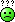#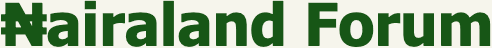Welcome, Guest: Join Nairaland / LOGIN! / Trending / Recent / New
Stats: 2,758,315 members, 6,556,221 topics. Date: Tuesday, 26 October 2021 at 12:20 AM

## I'll Send A Card Worth #2000 If Anyone Can Solve These Problems - Education - Nairaland

 I'll Send A Card Worth #2000 If Anyone Can Solve These Problems by sopstan(m): 5:50am On Jan 20, 2018 You will recieve 2k worth of airtime if you get these questions within 5hrs 1. integral[1/((e^t)-1)]dt 2. integral(lnx) 3. show that |n|n-1=π/sinnπ (| rep a sign in gamma and beta function) 4. show that beta(m,n)=(|p|q)/|(p+q) Re: I'll Send A Card Worth #2000 If Anyone Can Solve These Problems by dingbang(m): 5:53am On Jan 20, 2018 U dey find person wey go solve your assignment for you abi.. No worry...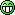Things will be made easy soon for you 3 Likes Re: I'll Send A Card Worth #2000 If Anyone Can Solve These Problems by doughziay: 5:56am On Jan 20, 2018 sopstan:You will recieve 2k worth of airtime if you get these questions within 5hrs 1. integral[1/((e^t)-1)]dt 2. integral(lnx) 3. show that |n|n-1=π/sinnπ (| rep a sign in alpha and beta function) 4. show that beta(m,n)=(|p|q)/|(p+q)I'll pay you double that amount if you'll solve it. 4 Likes Re: I'll Send A Card Worth #2000 If Anyone Can Solve These Problems by sopstan(m): 5:59am On Jan 20, 2018 ok after 5hrs...Re: I'll Send A Card Worth #2000 If Anyone Can Solve These Problems by presh2dk(m): 6:16am On Jan 20, 2018 Abeg keep your card Re: I'll Send A Card Worth #2000 If Anyone Can Solve These Problems by sopstan(m): 6:20am On Jan 20, 2018 presh2dk:Abeg keep your card you've tried your best right?...e pain am 1 Like Re: I'll Send A Card Worth #2000 If Anyone Can Solve These Problems by sopstan(m): 6:21am On Jan 20, 2018 you've tried your best right?...; e pain am 1 Like Re: I'll Send A Card Worth #2000 If Anyone Can Solve These Problems by LordSkinnie(m): 6:27am On Jan 20, 2018 Simple. Answer - South Africa.where my card?sopstan:You will recieve 2k worth of airtime if you get these questions within 5hrs 1. integral[1/((e^t)-1)]dt 2. integral(lnx) 3. show that |n|n-1=π/sinnπ (| rep a sign in alpha and beta function) 4. show that beta(m,n)=(|p|q)/|(p+q) 1 Like Re: I'll Send A Card Worth #2000 If Anyone Can Solve These Problems by dejt4u(m): 6:35am On Jan 20, 2018 sopstan:You will recieve 2k worth of airtime if you get these questions within 5hrs 1. integral[1/((e^t)-1)]dt 2. integral(lnx) 3. show that |n|n-1=π/sinnπ (| rep a sign in alpha and beta function) 4. show that beta(m,n)=(|p|q)/|(p+q)this resembles Mathematical Physics.. Beta and Gamma functions (Euler's function) Re: I'll Send A Card Worth #2000 If Anyone Can Solve These Problems by sopstan(m): 6:38am On Jan 20, 2018 dejt4u:this resembles Mathematical Physics.. Beta and Gamma functions (Euler's function) something like that Re: I'll Send A Card Worth #2000 If Anyone Can Solve These Problems by falcon01: 7:16am On Jan 20, 2018 MAKE I SOLVE Re: I'll Send A Card Worth #2000 If Anyone Can Solve These Problems by sopstan(m): 8:01am On Jan 20, 2018 sopstan:You will recieve 2k worth of airtime if you get these questions within 5hrs 1. integral[1/((e^t)-1)]dt 2. integral(lnx) 3. show that |n|n-1=π/sinnπ (| rep a sign in alpha and beta function) 4. show that beta(m,n)=(|p|q)/|(p+q)2. sol integral(Inx)= integral(lnx.x°) (where x°=1) this becomes integral(udv)u=Inx and dv=x°du=1/x and v=x then using integratn by parts you have uv-integral(vdu) which will beInx.x-integral(x.1/x)dx = xInx-x = x(Inx-1)ans...Re: I'll Send A Card Worth #2000 If Anyone Can Solve These Problems by Mrkumareze(m): 8:13am On Jan 20, 2018 It's like you don't know where to post such question. Nairaland is characterized by lazy men and women 2 Likes Re: I'll Send A Card Worth #2000 If Anyone Can Solve These Problems by sopstan(m): 8:32am On Jan 20, 2018 sopstan:You will recieve 2k worth of airtime if you get these questions within 5hrs 1. integral[1/((e^t)-1)]dt 2. integral(lnx) 3. show that |n|n-1=π/sinnπ (| rep a sign in alpha and beta function) 4. show that beta(m,n)=(|p|q)/|(p+q) 1. sol integral[1/((e^t)-1)]dtu=e^t, du=e^tdt, dt=du/e^t=du/uthe whole guy now becomes integral[du/u(u-1)] then you resolve into partial fraction it now becomes integral[1/(u+1)-1/u]du you then integrate=In(u+1)-Inu=In[(u+1)/u] (since u=e^t) the whole guy now becomesIn[((e^t)+1)/e^t] or In[1+1/e^t]ans...now do the rest Re: I'll Send A Card Worth #2000 If Anyone Can Solve These Problems by sopstan(m): 8:34am On Jan 20, 2018 Mrkumareze:It's like you don't know where to post such question. Nairaland is characterized by lazy men and women like seriously? Re: I'll Send A Card Worth #2000 If Anyone Can Solve These Problems by BunbleBee: 9:18am On Jan 20, 2018 3).Another common integral representation of the beta function isB( x , y ) = ∫ ∞ 0 t x −1(1 + t ) x + y dt .SoΓ( n)Γ(n-1) = B( n, n − 1) = ∫ ∞ 0 t n −11 + t dt .That integral can be evaluated by consideringf( z ) = z n −11 + z and integrating around a keyhole contour with the branch cut for z n-1 along the positive real axis.Then∫ ∞ 0 t n−11 + t dt + ∫ 0 ∞ ( te 2 πi ) n −11 + t dt = 2 πi Res[ f ( z ),= 2 πi ( e πi ) n −= − 2 πie πinwhich implies∫ ∞ 0 t n −11 + t dt = −2 πie πin1 − e 2 πin= π 2 ie πin − e − πin= πsinπn Re: I'll Send A Card Worth #2000 If Anyone Can Solve These Problems by sopstan(m): 9:36am On Jan 20, 2018 BunbleBee:3).Another common integral representation of the beta function isB( x , y ) = ∫ ∞ 0 t x −1(1 + t ) x + y dt .SoΓ( n)Γ(n-1) = B( n, n − 1) = ∫ ∞ 0 t n −11 + t dt .That integral can be evaluated by consideringf( z ) = z n −11 + z and integrating around a keyhole contour with the branch cut for z n-1 along the positive real axis.Then∫ ∞ 0 t n−11 + t dt + ∫ 0 ∞ ( te 2 πi ) n −11 + t dt = 2 πi Res[ f ( z ),= 2 πi ( e πi ) n −= − 2 πie πinwhich implies∫ ∞ 0 t n −11 + t dt = −2 πie πin1 − e 2 πin= π 2 ie πin − e − πin= πsinπnthe integral limit should be 0-1 since it's a beta function B(m,n) you try weldone Re: I'll Send A Card Worth #2000 If Anyone Can Solve These Problems by BunbleBee: 9:42am On Jan 20, 2018 . Re: I'll Send A Card Worth #2000 If Anyone Can Solve These Problems by MYSELF2018: 9:43am On Jan 20, 2018 sopstan:You will recieve 2k worth of airtime if you get these questions within 5hrs 1. integral[1/((e^t)-1)]dt 2. integral(lnx) 3. show that |n|n-1=π/sinnπ (| rep a sign in alpha and beta function) 4. show that beta(m,n)=(|p|q)/|(p+q) I can solve it for you. I am not with my android phone here, but I'l send it in the evening when I get back home. Please kindly send your whatsap number to praisejummy42@gmail.com Re: I'll Send A Card Worth #2000 If Anyone Can Solve These Problems by spartandude: 7:07am On Jan 21, 2018 which you championed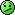Mrkumareze:It's like you don't know where to post such question. Nairaland is characterized by lazy men and women Re: I'll Send A Card Worth #2000 If Anyone Can Solve These Problems by spartandude: 7:10am On Jan 21, 2018 Anus ofcourse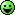sopstan: we're talking maths here this one is talking trash pls where do you think from